Get instant live expert help with Excel or Google Sheets“My Excelchat expert helped me in less than 20 minutes, saving me what would have been 5 hours of work!”

#### Post your problem and you’ll get expert help in seconds.

Your message must be at least 40 characters
Our professional experts are available now. Your privacy is guaranteed.

# How to Perform VLOOKUP Between Two Workbooks

Excel has a large number of functions to perform different tasks. VLOOKUP is one such function that is used for lookup and reference. Normally, VLOOKUP cannot lookup values across multiple workbooks. To perform lookup across multiple workbooks you have to nest an INDIRECT function inside a VLOOKUP and use an INDEX MATCH function. In this tutorial, we will show you step-by-step how to create a VLOOKUP formula across on multiple workbooks.

## Use VLOOKUP across Multiple Workbooks

The following example includes the Product ID and Name for different products. The data contains four workbooks for four different categories.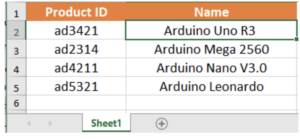arduino.xlsx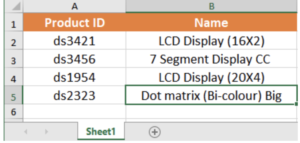display.xlsx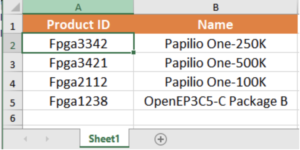fpga.xlsx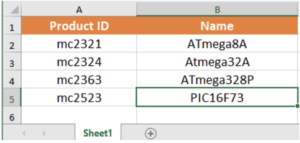micro_controller.xlsx

To find out the necessary products to complete a project based on the ID’s, you will use the VLOOKUP formula across these workbooks to extract the values. To do that:

1. Create a new sheet Project Requirements. In cells E2:E5, list the names of the four workbooks. Now in cell A2, put the ID that needs to be looked up. In cells F2, assign the formula `=IFERROR(VLOOKUP(\$B\$1,INDIRECT("["&\$E\$2:\$E\$5&"]Sheet1"&"!\$A\$2 :\$B\$5"), 2, FALSE),””)` and drag it down till cell F5.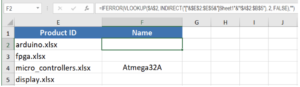1. Press Ctrl + Shift + Enter to apply the formula to F2. Drag the formula from F2 to F5.

1. Now in cell B2, assign the formula `=INDEX(F2:F5, MATCH(TRUE,NOT(F2:F5=""),0), 0).`
1. Press Ctrl + Shift + Enter to apply it to B2.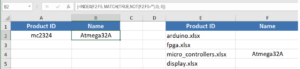This will return the product name for the corresponding product ID in A2 which belong to multiple workbooks. It uses the functions IFERROR, VLOOKUP and INDIRECT. The VLOOKUP performs the actual lookup based on the INDIRECT calls to the four workbooks. IFERROR is used to make sure the errors are handled. These functions work together in order to enable lookup across multiple workbooks. Thus you can look up across multiple workbooks and still use VLOOKUP.

VLOOKUP is one of the most effective functions to lookup values in Excel. Though VLOOKUP does not work with multiple workbooks, you saw in this tutorial how to use VLOOKUP with multiple workbooks using other functions.

Are you still looking for help with the VLOOKUP function? View our comprehensive round-up of VLOOKUP function tutorials here.

How to Use a VLOOKUP in Excel – Excelchat

How to Use a VLOOKUP in Google Sheets – Excelchat

VLOOKUP Not Working? (Find Out Why) – Excelchat

How to Use VLOOKUP with Multiple Criteria – Excelchat

How to Use VLOOKUP and IF Functions Together – Excelchat

INDEX MATCH versus VLOOKUP: How and When to Use – Excelchat

How to Use VLOOKUP across Multiple Sheets in Excel – Excelchat

### Did this post not answer your question? Get a solution from connecting with the expert.Another blog reader asked this question today on Excelchat:
Solution examplesI filtered data in column A (it is labeled ID #) of a data set. On my next worksheet, column A is also the ID #, but it is a different data set. I want to filter the ID #'s the same for the two sheets, but how do I do that being that I am working with two different sets of data?
Solved by V. H. in 22 minsI have two columns with names and a third one with email addresses. For every name that matches, I need to copy the email address to an empty column next to the matching name. .
Solved by E. U. in 15 minsI'm having trouble with an index & match formula where I'm looking up two different pieces of info in order to return a specific value.
Solved by K. D. in 54 minshi, my vlookup wont work in some rows but does in others. its temperemental. e.g it will work on rows 10 to 15, then not row 16 and 17, but the will for the rest.
Solved by K. Q. in 21 minsHow can I automatically change different cells and the information they display if we select different option from a drop down list?
Solved by V. U. in 59 mins## Subscribe to Excelchat.coAnother blog reader asked this question today on Excelchat: